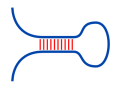Freiburg RNA Tools
Teaching - MEA# Teaching - MEA : max. exp. accuracy source at github@BackofenLab/RNA-Playground

To predict the structure with maximum expected accuracy (MEA) for a given RNA sequence, the algorithm introduced by Zhi J. Lu and co-workers (2009) uses the sequence's base pair and unpaired probabilities. The approach follows a Nussinov-like recursion using the probabilities derived from John S. McCaskill's algorithm.

Here, we use our simplified McCaskill approach for the probability computation. Therein we apply a Nussinov-like energy scoring scheme, i.e. each base pair of a structure contributes a fixed energy term $E_{bp}$ independent of its context.

Furthermore, beside the identification of an optimal MEA structure via traceback, we provide an exhaustive enumeration of up to 15 suboptimal structures using the algorithm by Stefan Wuchty et al. (1999). For each structure, the according traceback is visualized on selection.RNA sequence:
Minimal loop length $l$:
Energy weight of base pair $E_{bp}$:
'Normalized' temperature $RT$:
Base pair weighting $\gamma$:
Delta to MEA:

## MEA structure prediction

The MEA structure predictions uses the following recursion to fill a dynamic programming table $M$. An entry $M_{i,j}$ provides the MEA score for the subsequence $S_{i}..S_{j}$, such that the overall score is found in $M_{1,n}$ for a sequence of length $n$.
Possible Structures
Select a structure from the list or a cell of $M$ to see according tracebacks. Note, the structure list is limited to the first 15 structures identified via traceback.
Below, we provide a graphical depiction of the selected structure. Note, the rendering does not support a minimal loop length of 0.
Visualization done with forna. Base pairs are given by red edges, the sequence backbone is given by gray edges.

## Probabilities used

Given the partition functions $Q$ and $Q^{bp}$ provided by the McCaskill algorithm, we can compute the probabilities of individual base pairs $(i,j)$ within the structure ensemble, i.e. $P^{bp}_{i,j} = \sum_{P \ni (i,j)} \exp(-E(P)/RT) / Z$ given by the sum of the Boltzmann probabilities of all structures that contain the base pair. For its computation, the following recursion is used, which covers both the case that $(i,j)$ is an external base pair as well as that $(i,j)$ is directly enclosed by an outer base pair $(p,q)$.
$P^{bp}$

The following formula is used to compute the probability $P^u_{i}$ that a given sequence position $S_{i}$ is not paired. The probabilities are directly inferred from the base pair probabilities $P^{bp}$.
$P^u$